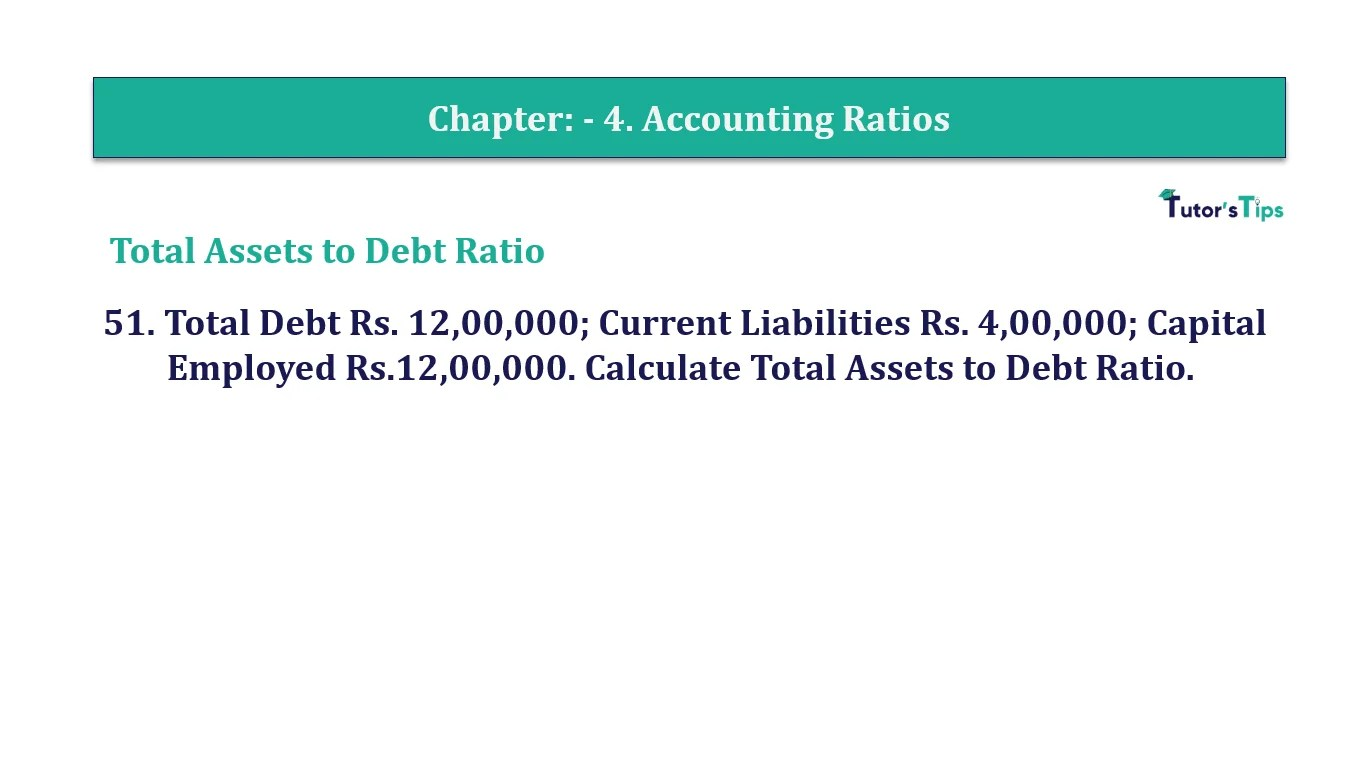# Question 51 Chapter 4 of +2-B – T.S. Grewal 12 ClassQuestion No. 51- Chapter No.4 - T.S. Grewal +2 Book Part B

Question 51 Chapter 4 of +2-B

Total Assets to Debt Ratio

51. Total Debt Rs. 12,00,000; Current Liabilities Rs. 4,00,000; Capital
Employed Rs.12,00,000. Calculate Total Assets to Debt Ratio.

### The solution of Question 51 Chapter 4 of +2-B: –

 Debt = Total Debts – Current Liabilities = Rs. 12,00,000 – Rs. 4,00,000 Debt = Rs. 8,00,000 Total Assets = Capital Employed + Current Liabilities = Rs. 12,00,000 – Rs. 2,20,000 Long-term Debt = Rs. 16,00,000

 Total Assets to Debt Ratio = Total Assets = Rs.16,00,000 Long- term Debts Rs. 8,00,000 = 2: 1

Balance Sheet: Meaning, Format & Examples

Comment if you have any question.

Also, Check out the solved question of previous Chapters: –